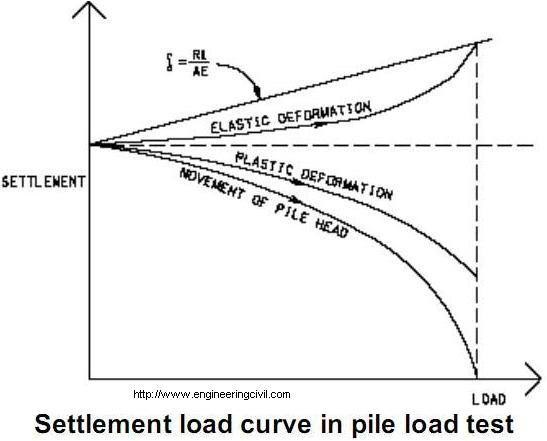Search

# What is the purpose of conducting load test for piling works?

Posted in Piles Engineering |Email This Post |

Pile load test provides information on ultimate bearing capacity but not settlement behavior. In essence, it can determine if the load is taken up by the stratum designed or if the centre of resistance is at the design location in piles as suggested by Robert D. Chellis (1961).

After conducting load tests, the curve of movement of pile head (Settlement against load) and the curve of plastic deformation can be plotted. By subtracting the curve of plastic deformation from the curve of pile head movement at each load, the curve of elastic deformation can be obtained. For piles of end-bearing type unrestrained by friction, the theoretical elastic deformation can be calculated from

e=RL/AE where e is

elastic deformation,
L is pile length,
A is area of pile,
E is Young’s Modulus of pile material
R is the reaction load on pile.

By substituting e in the formula, the elastic deformation read from the curve of elastic deformation, L can be obtained which shows the location of the centre of resistance corresponding to that load.This question is taken from book named – A Self Learning Manual – Mastering Different Fields of Civil Engineering Works (VC-Q-A-Method) by Vincent T. H. CHU.

More Entries :